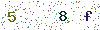﻿ LEDs Test Method: LED Color Measurement 2 - LISUNLISUN

# LEDs Test Method: LED Color Measurement 2

3 Spectrophotometric Method for Measuring the Chromaticity Coordinates.

According to method 2005, measure the spectrum power distribution P (λ) curve, then calculate the chromaticity coordinates of the measured device.

4 Photo electricity Integral Method for Measuring the Chromaticity Coordinates

Revise the spectrum response optical system of the photoelectric detector to comply with the spectrum stimulus value of the CIE 1931 standard photometric observer. This kind of light detection system can do one-time integral measurement of the three stimulus value of the optical radiation of the device under test and calculate the chromaticity coordinates.

5 Dominant wavelength

Any one color can be seen as the color mixed with one spectrum color and a reference illuminant (such as CIE standard illuminant A, B, C, light source E, standard illuminant D65), according to a certain proportion.

This spectrum color is the main wavelength of the color. The main wavelength is equivalent to the color tone (psychological quantity) that the human eye can observe.

If the chromaticity coordinates of the measured LED device is known, the equal energy white light E, the light source（ x0＝0.3333，y0 ＝0.3333）can be taken as the main wavelength to calculate and determine the color. According to the slope of the line in the chromaticity diagram that connects the reference illuminant chromaticity points and the sample color chromaticity points, calculate and read out the point of intersection of the line and the spectrum to determine the main wavelength.

6 Purity

The degree of the sample color close to the spectral color of the main wavelength indicates the purity of the sample color. In the CIE－1931 chromaticity diagram, the purity refers to the ratio of the distance from the online reference illuminant chromaticity point to the sample chromaticity point and the distance from the reference illuminant chromaticity point to the spectrum chromaticity points. The purity of the color is basically in line with the color observed by the human eyes.

7 Tc Color temperature

Source of the relative spectrum power distribution that deviates from the farther blackbody relative spectrum power distribution; we use the chromaticity coordinate and the nearest blackbody to indicate the relative color temperature ( Color Temperature Meter ) of the light source, and calculate the relative color temperature.